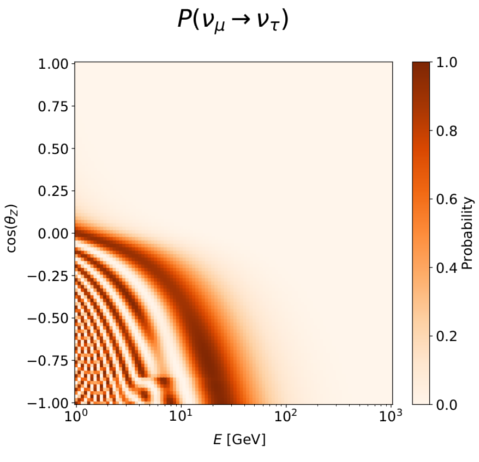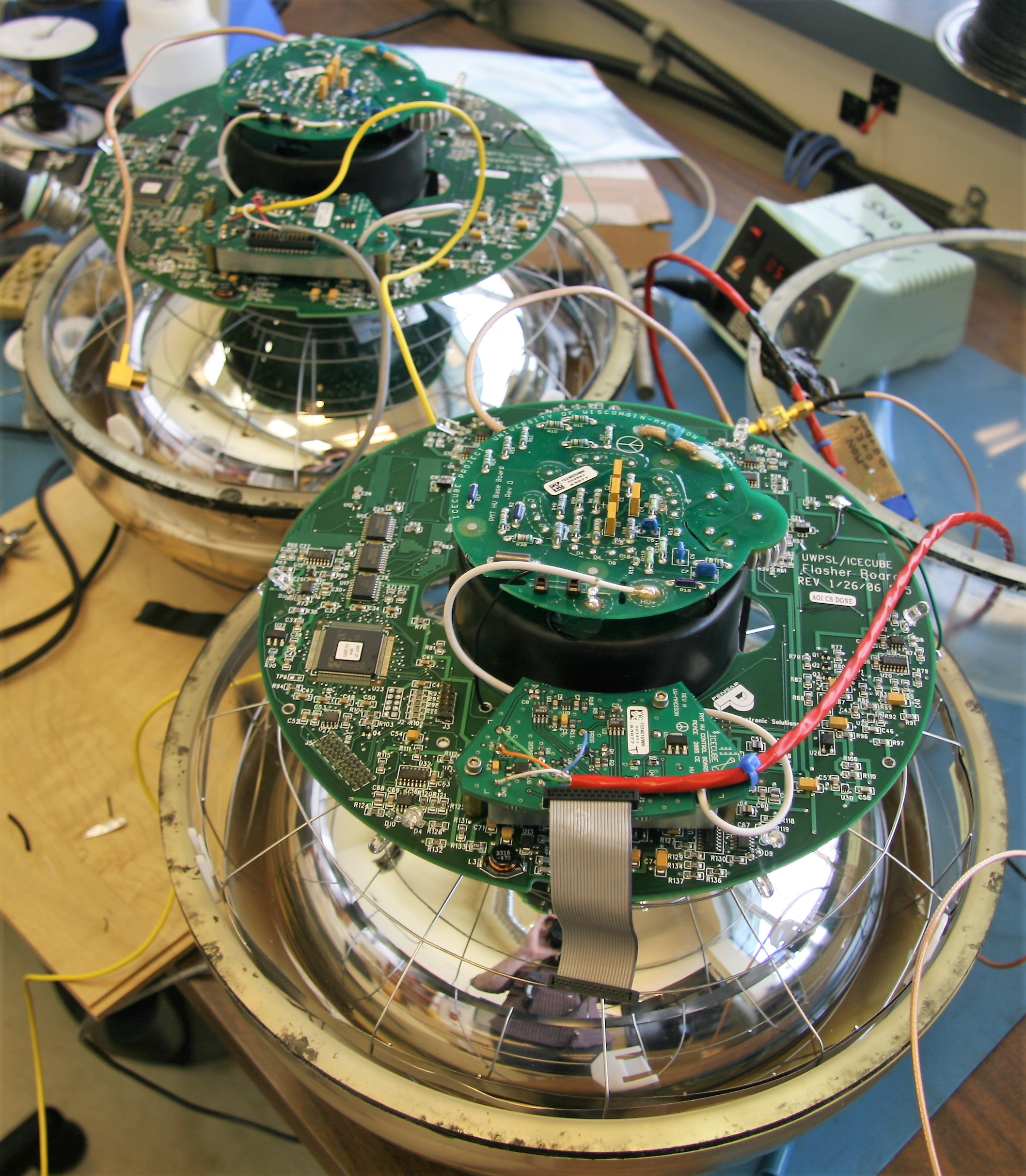# Neutrino oscillation

In addition to high-energy neutrinos of astrophysical origin, IceCube detects thousands of lower-energy neutrinos produced by the interaction of cosmic rays with the Earth's atmosphere. This high-statistics sample of neutrinos can be a useful probe for studying properties of these particles, like neutrino oscillation.

The discovery of neutrino oscillation is a relatively new accomplishment, which was awarded the Nobel prize in physics in 2015. Oscillation refers to a puzzling behaviour that neutrinos have, whereby neutrinos produced as one flavour may be detected as another.

The current theoretical understanding of neutrino oscillation is that the flavour states of the neutrinos are actually a superposition of mass eigenstates. The probability of a neutrino to oscillate into another flavour state can be described by a 3x3 matrix called the PMNS matrix. Assuming this matrix is unitary, it can be fully defined by 3 mixing angles and a CP-violating phase.Oscillation probability plot of a muon neutrino reaching IceCube. Depending on its zenith angle, a neutrino produced in an air shower will travel along different baselines through the Earth. Thus, its probability for oscillating into a given flavour will depend on its initial energy (the horizontal axis of this plot), and its effective "L" (represented by the measured zenith direction in IceCube, on the vertical axis). One can see that the probability of the muon neutrino to convert into a tau neutrino is close to 1.0 at various bands of the L/E phase space IceCube is sensitive to.

For a detector like IceCube, the probability of an atmospheric neutrino being detected as a different flavour depends on the distance travelled by the neutrino and its energy. By observing neutrino oscillations in IceCube, the fundamental properties of neutrinos such as the mixing angles and the mass differences between different eigenstates can be measured.

## IceCube-DeepCore

To study low-energy neutrino interactions (which appear dimmer in the detector than high-energy neutrinos), a set of 8 detector strings more densily instrumented was installed at the center of the IceCube detector. These strings form the basis of the DeepCore array, and allow us to significantly lower the energy threshold above which it is possible to observe neutrinos to approximately 10 GeV.

## Statistical appearance of the tau neutrino

A major focus of our group concerns the oscillation-induced appearance of the tau neutrinos in the IceCube neutrino data. In atmospheric showers, muon neutrinos are abundantly produced. For muon neutrinos travelling through the Earth from the northern hemisphere to DeepCore, the distance they travel is optimal for them to be detected as tau neutrinos.

Our group is measuring the number of upward-going tau neutrino events observed in the DeepCore detector, and comparing it to the expected number of tau neutrinos that should appear under a typical, three-flavours oscillation scenario. Significant divergence from the expected count of tau neutrinos could indicate limitations in our current understanding of neutrinos, thus laying experimental evidence for new physics.

## Neutrino Environmental Decoherence

It has been postulated that as neutrino states propagate through space-time, they may weakly couple to their environment and in doing so share quantum information with this environment. Phenomena of this kind is predicted by some quantum gravity models. This coupling to the environment modfies the oscillation properties of the neutrinos compared to the standard picture, and a search is underway by our group to identify such an effect in the neutrinos observed by the DeepCore detector.

## Calibration studiesThe dark noise structure of the IceCube DOMs has been the focus of many studies. The current model of this noise implemented in all IceCube analyses has been developed by one of our graduate students.

The lower energies of the neutrinos used in oscillation analyses also mean that the luminosity of the events considered in the detector is much less than that of very-high-energy, astrophysical neutrinos. This means that the main source of uncertainty in the low-energy studies are systematic uncertainties that come from our understanding of our detector, and of the light propagation process in glacial ice. Our group is involved in the modeling of dark noise within the IceCube Digital Optical Modules (DOMs), and in the development of novel techniques to better measure local optical properties of the ice between individual sensors.